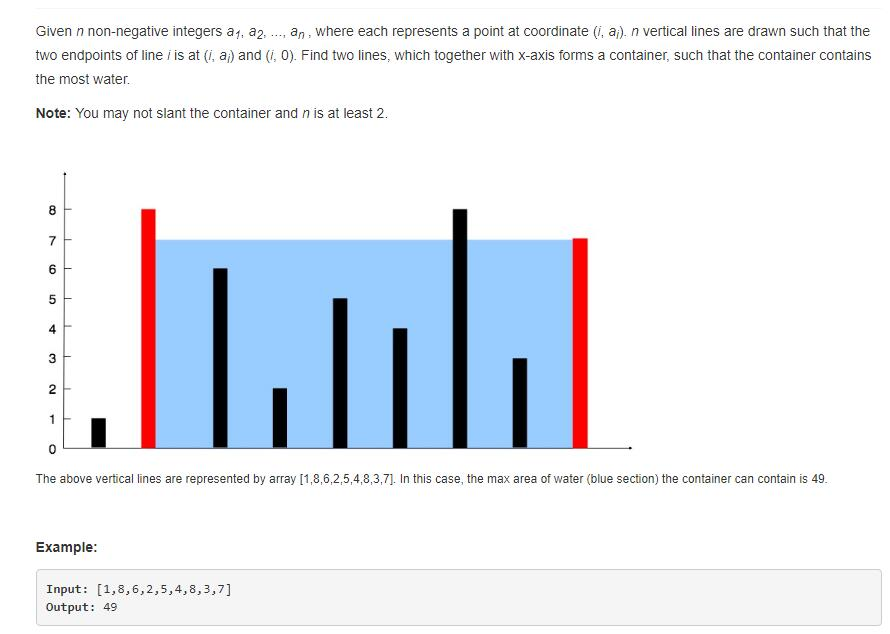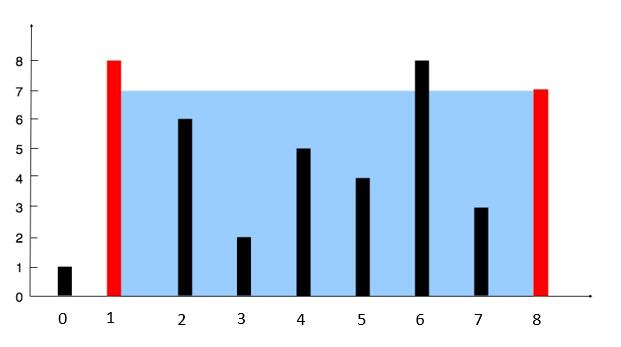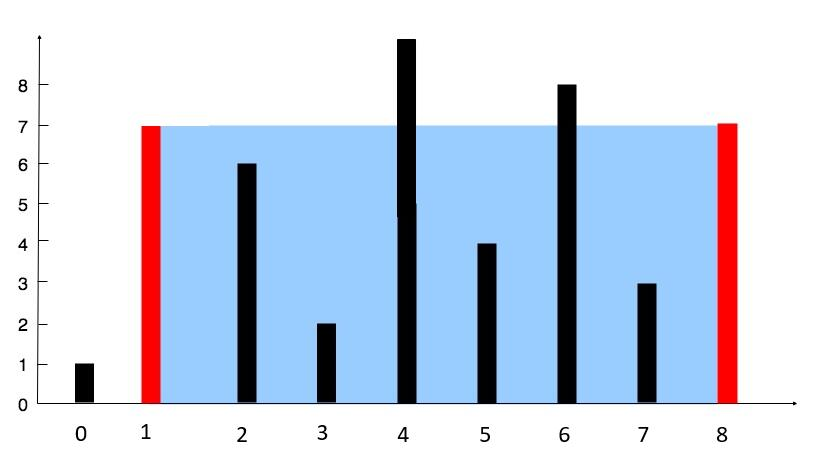# 题目描述（中等难度）# 解法一 暴力解法

public int maxArea(int[] height) {
int max = 0;
for (int i = 0; i < height.length; i++) {
for (int j = i + 1; j < height.length; j++) {
int h = Math.min(height[i], height[j]);
if (h * (j - i) > max) {
max = h * (j - i);
}
}
}
return max;
}


# 解法二public int maxArea2(int[] height) {
int maxarea = 0, l = 0, r = height.length - 1;
while (l < r) {
maxarea = Math.max(maxarea, Math.min(height[l], height[r]) * (r - l));
if (height[l] < height[r])
l++;
else
r--;
}
return maxarea;
}